﻿ On an Integer Sequence via Euler <i>ϕ</i> FunctionPublications are Open
Access in this journal
Article Versions
Export Article
• Normal Style
• MLA Style
• APA Style
• Chicago Style
Research Article
Open Access Peer-reviewed

### On an Integer Sequence via Euler ϕ Function

Binu K P, Vinod S, K Vishnu Namboothiri
American Journal of Applied Mathematics and Statistics. 2019, 7(3), 112-114. DOI: 10.12691/ajams-7-3-5
Received February 11, 2019; Revised March 20, 2019; Accepted May 08, 2019

### Abstract

Similar to Fibonacci sequence and Lucas sequences that are recursively defined we define an integer sequence using the Euler totient function ϕ and study some of its properties. We also verify that the sequence we have defined has some properties similar to Fibonacci sequence, but even then it is not a Lucas sequence.

### 1. Introduction

There have been various attempts in the recent literature to study properties related to integer sequences similar to Fibonacci sequence which is defined recursively by F0 = 1, F1 = 1 and Fn = Fn-1 +Fn-2 for n> 1. Fibonacci sequence has many interesting properties like even being consisting of very large terms after a few number of initial terms, the ratio of consecutive terms converges to a finite number. Another interesting property of Fibonacci sequence is that it has no perfect numbers in it (See 1). A larger class of sequences is defined generalizing the Fibonacci sequence called Lucas sequences. They also share some of the important properties of Fibonacci sequences. In this article, we define an integer sequence via the arithmetical function. Euler ϕ function and try to obtain some properties of this sequence contrasting with the Fibonacci sequence

### 2. Notations and Basic Results

In what following, ϕ will denote the Euler totient function. Therefore, ϕ (n) is the number of positive integers < n that are co prime to n, n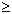1. By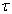(n), we mean the number of divisors of n.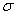(n) will denote the sum of divisors of n including n itself. n is said to be perfect if(n) = 2n. Some examples for perfect numbers are 6 and 28.

Now we state a classical result on convergence of real numbers called as the Stolz-Cesaro theorem:

Theorem 2.1. [ 3, Chapter 3, Theorem 1.2.2]

Let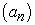be a sequence of real numbers and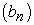a strictly monotone and divergent sequence. Then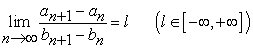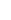Implies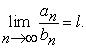The Fibonacci sequence, as we mentioned above in the introduction is defined recursively as F0 = 1, F1 = 1 and Fn = Fn-1 + Fn-2 for n2. For a fixed pair of integers (p, q), Lucas sequence is defined as choosing l0, l1 arbitrary, and defining ln = pln-1 qln-2. If we let p = 1 and q = -1, it becomes the definition of the Fibonacci sequence.

It is known that Fibonacci sequence or Lucas sequence do not contain any perfect numbers 1.

### 3.ϕ-Integer Sequences and Some of Its Properties

We now state a lemma which we are going to use frequently.

Lemma 3.1. The ϕ function satisfies the following bounds:

(1)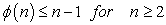(2)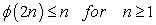(3)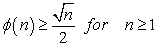Proof. The statement (1) is obvious. To see (2) note that for every 2n, half of the number of numbers below or equal to that are even and so they are not co-prime to 2n. Hence a maximum of n of them only can be co-prime to 2n. Hence statement (2) follows. The claim in (3) has been established by J. Browkin while trying to see the weaker result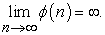This proof can be found, for example in [ 4, Chapter 6, Theorem 4].

We now define a sequencerecursively as follows. Take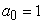. Define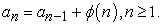Some initial values of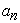are 1, 2, 3,5, 7, 11, 13, 19, 23, 29, 33 . . . This sequence is clearly a monotone increasing sequence and goes to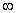with n. Let us call it as the phi-sequence. This sequence is in fact called as the totient summatory function in the literature. We now showcan be restricted above by some polynomial in n. We summarize our claims in the next two propositions.

Proposition 3.2.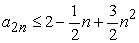for every n.

Proof. By definition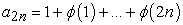. We now split this over even and odd indices. Thus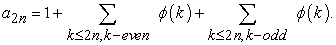If k is even, then by lemma (3.1),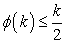and if k odd (or in fact for any k),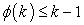. Then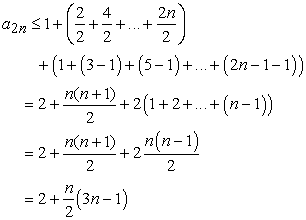which is what we claimed.

Proposition 3.3.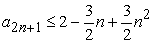for every n.

Proof. Note that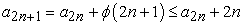. Now use the bound obtained in the above proposition to conclude.

Fibonacci sequence is monotonically increasing to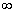with n. One of the key properties of Fibonacci sequence is that the ratio of consecutive terms converges to the number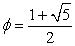= 1.6180339887 …. Though the terms are increasing by large leaps, the ratio of terms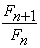surprisingly approaches a finite number. In the next result, we show that the sequence we defined above has the same property. That is, the limit of ratio of terms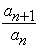converges to a finite number.

Theorem 3.4. The sequence of ratios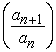converges to 1 as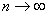.

Proof. Since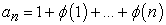we have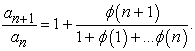By (1) and (3) in lemma (3.1),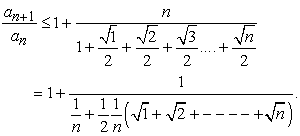Write xn =√1 +√2+…+√n and yn= n .Then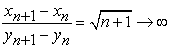by theorem (2.1), we have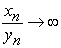Thusis bounded above by a sequence which converges to 1. Also, since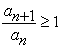by definition, it is bounded below by the constant sequence (1). Hence by squeeze theorem for real number sequences,also converges to 1.

We now prove that the sequence we have defined is not a Lucas sequence.

Theorem 3.5. There do not exist integers r, s such that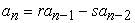which works for every n. In other words no tail of the ϕ sequence is Lucas.

Proof. On the contrary, suppose such r, s exists. Then since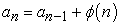we get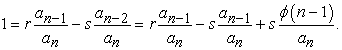Now the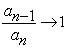. Since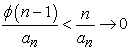as can be seen in the proof of theorem (3.4). So aswe get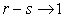. Since r, s fixed integers, r - s = 1. Thus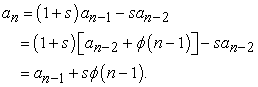Therefore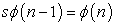. Note that s is positive by this equation. If we let n - 1 to be a prime, then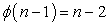. Since n is even,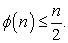If we take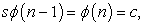this means that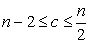, which is not true for n > 4. So such an s cannot exist. The infinitude of primes proves our claim.

We have already seen that Lucas sequences do not contain perfect numbers. Though we are unable to state such a result for our phi-sequence, we have experimental evidence to believe that such a statement holds for our sequence as well. We have tested it for all terms up to 106 (with the support of SageMath software) and could not locate a single perfect number. So we state it as a conjecture and leave it for future studies:

Conjecture 3.6. The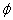sequence does not contain any perfect numbers.

There are many other questions one may find interesting with these type of sequences. For example, the set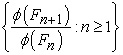is dense in the set of all positive numbers (see 2). Such a similar result can be discussed for our phi-sequence also. Further, we believe that similar properties may hold if we modify our sequence replacing the phi-function withor. We plan to discuss these sequences in a sequel to this article.

### References

  Florian Luca. Perfect fbonacci and lucas numbers. Rendiconti del Circolo Matematico di Palermo, 49(2):313-318, 2000. In article View Article  Florian Luca, V Janitzio Mejia Huguet, Av San Pablo, and Florin Nicolae. On the euler function of fbonacci numbers. Journal of Integer Sequences, 12(2):3, 2009. In article  Marian Muresan. A concrete approach to classical analysis, volume 14. Springer, 2009. In article View Article  Waclaw Sierpinski. Elementary Theory of Numbers: Second English Edition (edited by A. Schinzel), volume 31. Elsevier, 1988. In article

Published with license by Science and Education Publishing, Copyright © 2019 Binu K P, Vinod S and K Vishnu NamboothiriThis work is licensed under a Creative Commons Attribution 4.0 International License. To view a copy of this license, visit http://creativecommons.org/licenses/by/4.0/